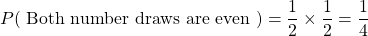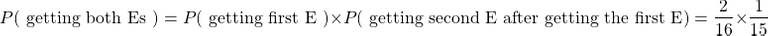# Probability - Sampling With Replacement And Without Replacement

in STEMGeeks3 years ago

Hello there. In this post I want to cover the topic of sampling with replacement and sampling without replacement from probability and statistics.

This topic is important when it comes to computing some probabilities and with statistical sampling.Pixabay Image Source

## Topics

• Dealing With Multiple Random Selections
• Sampling With Replacement
• Sampling Without Replacement Probability Examples

## Dealing With Multiple Random Selections

There are times when we have to randomly select items from a bunch or select a few people at random from a group. The random selection of items or people from a group is called a sample.

Sampling with replacement occurs when we select items from a group and the number of items from a group stays the same after each selection. An example of this is choosing three random digits from 0 to 9 to create a random three digit number. I can have 777 where the 7 repeats.

Sampling without replacement occurs when we select items from a group where the number of items from the group does not stay the same after each selection. Going back to the random digits example, if no repeated digits are allowed in create a three digit random number then we have sampling without replacement. An example of a three digit number for this case would be 149.

Another example for sampling without replacement is picking marbles randomly out of a bag. Suppose there are 10 marbles where 4 are red, 3 are blue and 3 are green.

You select two marbles at random without putting any of the marbles back in the bag. Not putting any of the marbles back in the bag is a case of sampling without replacement. This changes the number of marbles left in the bag after a marble is drawn. Picking out the first marble leaves 9 marbles in the bag and picking out the second marble leaves out 8 marbles left in the bag. If the marbles are put back in the bag after a marble is drawn, then that is a case of sampling with replacement.Pixabay Image Source

## A Probability Example On Sampling With Replacement

Let's say that Peter wants to randomly two numbers from 1 to 10 (inclusive). Repeats are allowed. What is the probability of Peter randomly choosing two numbers that are even numbers?

Because repeats are allowed this makes it a sampling with replacement case. The even numbers from 1 to 10 are 2, 4, 6, 8 and 10 which is 5 out of 10. There are five odd numbers out of ten which are 1, 3, 5, 7 and 9.

In a single random pull of a number there is a 50% or 0.5 chance of pulling an even number. The probability of an even number being randomly chosen in the first pick is 50%. After the first pull of the number, getting that same number is possible on the second random number pull as repeats are allowed. The probability of getting an even number on the second pull is also 50%.

The two number pulls are not related to each other as the first number choice does not affect the number of choices in the second number pull. This is an example of the concept of independence in probability. The probability of Peter randomly choosing two numbers that are both even numbers would be the product of the two halves.Pixabay Image Source

## Sampling Without Replacement Probability Examples

In this section, we cover a few examples where there is sampling without replacement (no independent events).

Example One - Letter Tiles

Consider the table below for letters from letter tiles and their counts. There are 16 letter tiles.

LetterCount
B3
D4
E2
S1
T5
M1

You randomly select two letter tiles from a bag. No letter tile is put back into the bag. What is the probability of getting both Es?

The probability of obtaining the first E is 2 out of 16. This is because there are two Es available from the total of 16. With the second E keep in mind that one letter tile is gone which reduces the available letter tiles from 16 to 15. Before the second letter pull there is only one E left. The probability of obtaining the second E after getting the first E in the first pull is 1 out of 15.

The probability of getting both Es is the product of the probability of getting the first E with the probability of getting the second E.Note that the probability of getting the second E after getting the first E is an example of a conditional probability. This conditional probability is dependent on actually obtaining the first E on the first letter pull.

Example Two - Card Picking

Three cards are randomly drawn from a standard deck of 52 cards. What is the probability of all 3 cards being both sevens?

In a standard deck of 52 cards there are four sevens. The probability of obtaining three sevens from three random draws is a product of the probability of getting a seven in the first draw with the probability of getting a seven in the second draw and the probability of getting a seven after the first two draws are sevens.Pixabay Image Source